#### How to Write Name with Initials

There were many times when those initials made me crazy. In this article, you can teach yourself how to handle initials with Excel. A few tricks for you here.

A few tricks for you on how to handle initials within Excel. All of them are combined in this article.

## Getting initials from a name

Let’s start with the easiest one. When only two-lettered initials are needed, just use:

=LEFT(\$A1)&MID(\$A1,FIND(” “,\$A1)+1,1)

This formula will give you the initials of the first name and surname. Two letters only.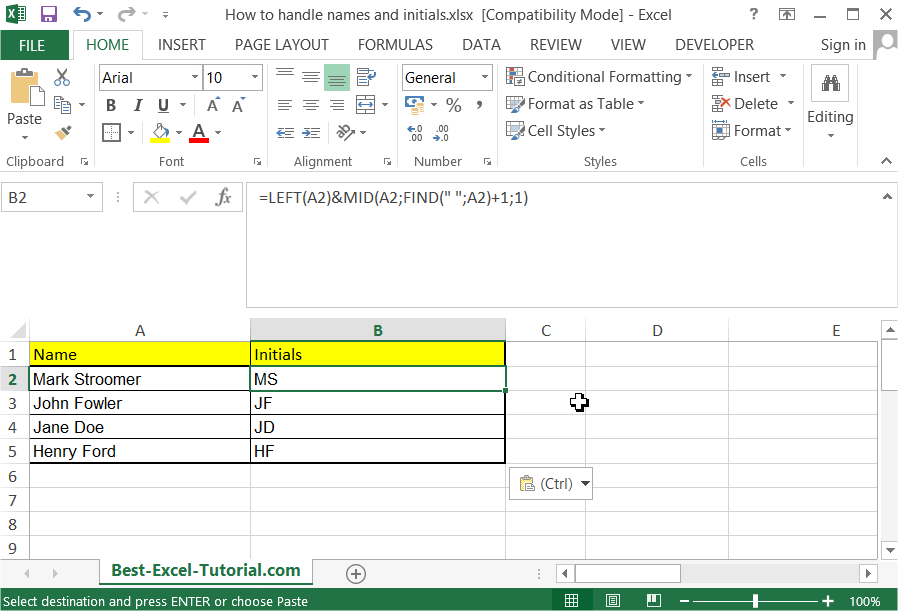### Getting initials from a name using CONCATENATE function

As an alternative you can use the CONCATENATE function as well:

1. First, enter the first name, middle initial, and last name into separate cells. For example, if the first name is “John”, the middle initial is “D”, and the last name is “Doe”, you would enter “John” into cell A1, “D” into cell B1, and “Doe” into cell C1.
2. In a new cell, enter the formula =CONCATENATE(A1,” “,B1,”. “,C1). This formula will combine the contents of cells A1, B1, and C1 into a single string, separated by spaces.
3. Press Enter to see the result. The name with initials should appear in the new cell, in the format “John D. Doe”.

You can also write the name with initials using the “&” operator instead of the CONCATENATE function, by entering the formula =A1&” “&B1&”. “&C1 into the new cell. The result should be the same as with the CONCATENATE function.

If you need to write the name with initials for a large number of names, you can use the same formula in multiple cells, copying it down to create a list of names with initials.

### Getting initials from a name using Text to Columns feature

Another method to write a name with initials in Microsoft Excel is to use the Text to Columns feature. This method is useful if you have a list of names in a single column, and you want to separate them into separate columns for the first name, middle initial, and last name. Here’s how:

1. Select the column that contains the names you want to separate.
2. Go to the Data tab on the ribbon and click on the Text to Columns button in the Data Tools section.
3. In the Convert Text to Columns Wizard, select the Delimited option and click Next.
4. Select the Space option under Delimiters and click Next.
5. In the next step, select the First Name, Middle Initial, and Last Name columns, and specify the appropriate data format for each column.
6. Click Finish to separate the names into separate columns.
7. In a new column, enter the formula =A1&” “&B1&”. “&C1, where A1, B1, and C1 contain the first name, middle initial, and last name respectively.
8. Press Enter to see the result. The name with initials should appear in the new cell, in the format “John D. Doe”.

You can then copy this formula down to the rest of the cells in the new column to create a list of names with initials.

## Getting initials from a first name, middle name, and surname

More advanced one. Use this one when the first name, middle name, and surname are always provided. Below, one will help you:

=LEFT(\$A1)&MID(\$A1,FIND(” “,\$A1)+1,1)&MID(RIGHT(\$A1,LEN(\$A1)-FIND(” “,\$A1,1)),FIND(” “,RIGHT(,LEN(\$A1)-FIND(” “,\$A1,1)))+1,1)

This formula gives you three initial letters. It will not work when there is no middle name provided.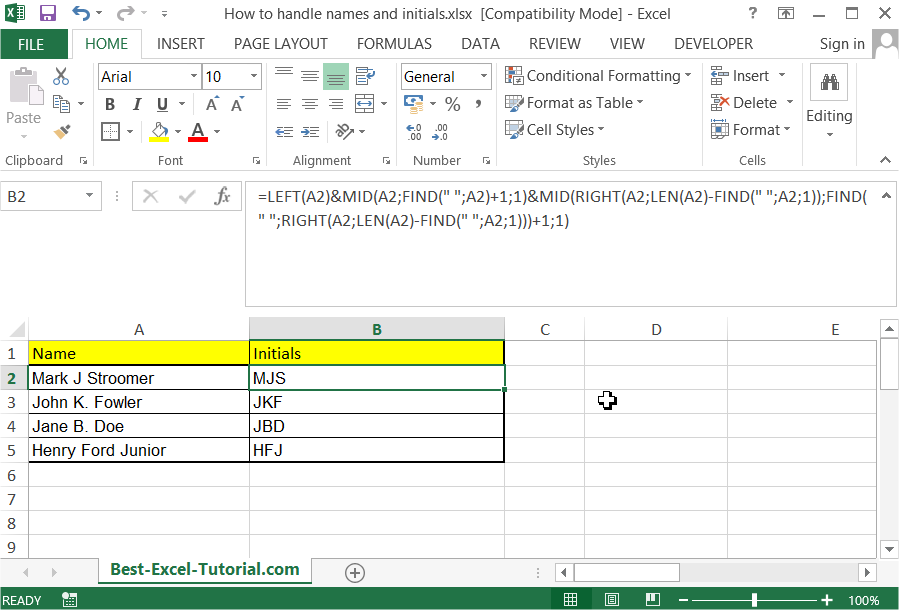### Getting initials from a first name, middle name, and surname using SUBSTITUTE and LEFT functions

Another method to get initials from a first name, middle name, and surname in Microsoft Excel is to use the SUBSTITUTE and LEFT functions. Here’s how:

1. Enter the first name, middle name, and surname into separate cells. For example, if the first name is “John”, the middle name is “David”, and the surname is “Doe”, you would enter “John” into cell A1, “David” into cell B1, and “Doe” into cell C1.
2. In a new cell, enter the formula =LEFT(A1,1)&SUBSTITUTE(B1,” “,””)&LEFT(C1,1).
3. Press Enter to see the result. The initials should appear in the new cell, in the format “JDD”.

This formula uses the LEFT function to extract the first character of the first name and surname. The SUBSTITUTE function is used to remove any spaces from the middle name and then take the first letter. The CONCATENATE function is then used to join these characters together into a single string.

## Getting initials from a full name

This is a complex formula which you can use to extract initials no matter what exactly you have in your data.

=IF(LEN(\$A1)-LEN(SUBSTITUTE(\$A1,” “,””))=0,LEFT(\$A1,1),IF(LEN(\$A1)-LEN(SUBSTITUTE(\$A1,” “,””))=1,LEFT(\$A1,1)&MID(\$A1,FIND(” “,\$A1)+1,1),LEFT(\$A1,1)&MID(\$A1,FIND(” “,\$A1)+1,1)&MID(\$A1,FIND(” “,\$A1,FIND(” “,\$A1)+1)+1,1)))

This formula is complicated but works every time.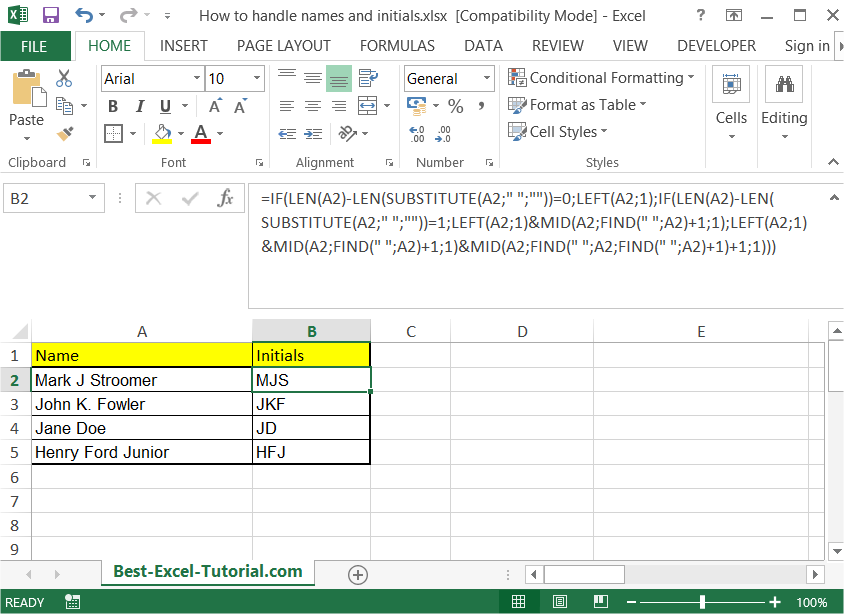### Getting initials from a full name using LEFT, RIGHT, and SEARCH functions

Another method to get initials from a full name in Microsoft Excel is to use the LEFT, RIGHT, and SEARCH functions along with the CONCATENATE function. Here’s how:

1. Enter the full name into a cell. For example, if the full name is “John David Doe”, you would enter “John David Doe” into cell A1.
2. In a new cell, enter the formula =LEFT(A1,1)&LEFT(RIGHT(A1,LEN(A1)-SEARCH(” “,A1,1)),1)&LEFT(RIGHT(A1,LEN(A1)-SEARCH(” “,A1,SEARCH(” “,A1,1)+1)),1).
3. Press Enter to see the result. The initials should appear in the new cell, in the format “JDD”.

This formula uses the LEFT function to extract the first character of the full name, the SEARCH function to locate the first and second spaces in the name, and the RIGHT function to extract the characters that come after each space. The CONCATENATE function is then used to join these characters together into a single string.

## Getting the first name and surname (without a middle name)

Use the below formula when you don’t care about middle names.

=LEFT(\$A1,SEARCH(” “,\$A1))&RIGHT(\$A1,LEN(\$A1)-SEARCH(” “,\$A1,SEARCH(” “,\$A1)+1))

This formula simplifies your data and cuts off middle names.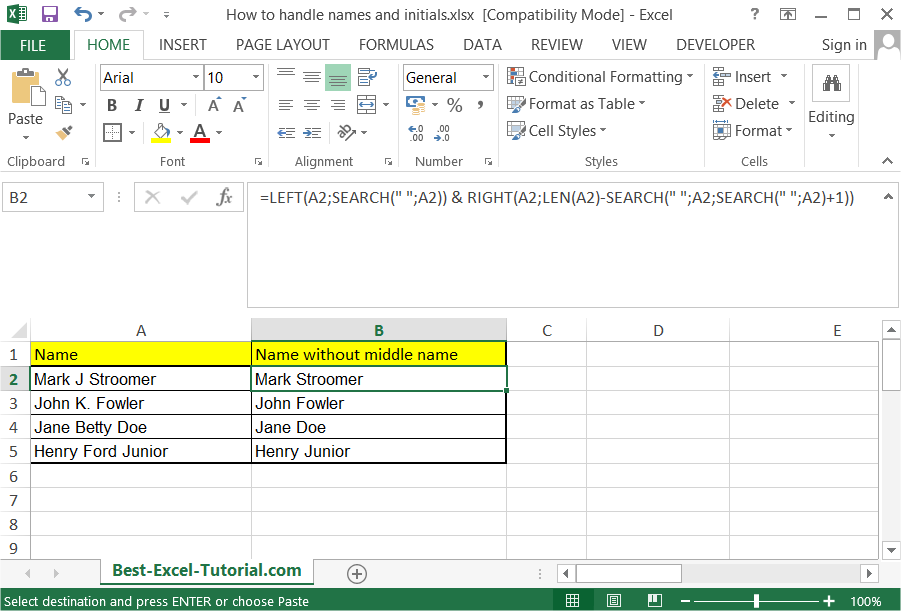### Getting the first name and surname (without a middle name) using LEFT, RIGHT and FIND functions

To extract the first name and surname (without a middle name) from a full name in Microsoft Excel, you can also use the LEFT and RIGHT functions along with the FIND function. Here’s how:

1. Enter the full name into a cell. For example, if the full name is “John Doe”, you would enter “John Doe” into cell A1.
2. In a new cell, enter the formula =LEFT(A1,FIND(” “,A1,1)-1) to extract the first name.
3. In another new cell, enter the formula =RIGHT(A1,LEN(A1)-FIND(” “,A1,1)) to extract the surname.
4. Press Enter to see the results. The first name should appear in the first new cell, and the surname should appear in the second new cell.

This formula uses the FIND function to locate the first space in the name and the LEFT and RIGHT functions to extract the characters that come before and after the space, respectively.

## Changing order of first name, middle name and surname

Thanks to this formula, you can change the order. In my example, you will get: surname coma space first name initial of middle name

=RIGHT(\$A1,LEN(G2)-FIND(“.”,\$A1)-1)&”, “&LEFT(\$A1,FIND(“.”,\$A1))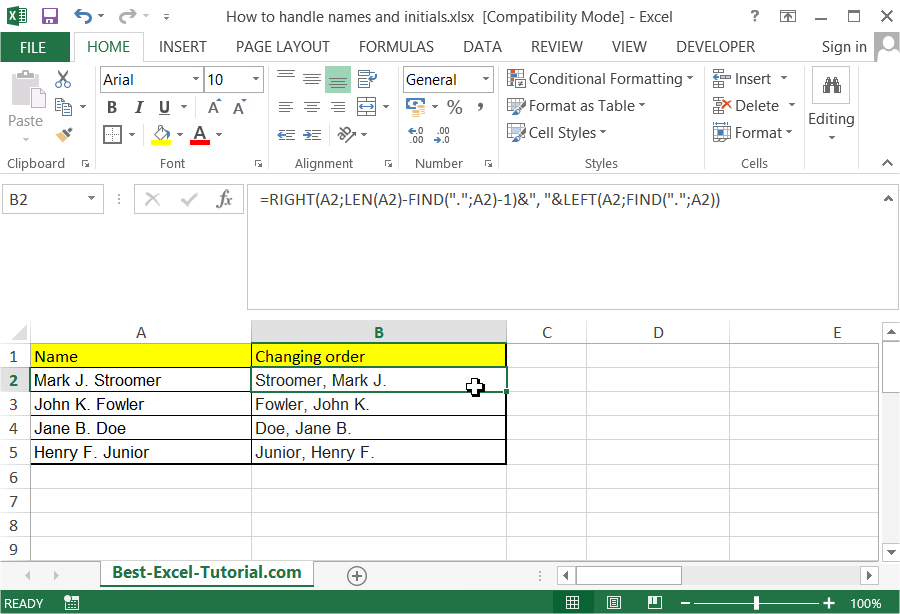### Changing order of first name, middle name and surname using LEFT, RIGHT, and MID functions

To change the order of the first name, middle name, and surname in Microsoft Excel, you can also use the LEFT, RIGHT, and MID functions along with the FIND function. Here’s how:

1. Enter the full name into a cell. For example, if the full name is “John David Doe”, you would enter “John David Doe” into cell A1.
2. In a new cell, enter the formula =RIGHT(A1,LEN(A1)-FIND(” “,A1,FIND(” “,A1,1)+1))&” “&MID(A1,FIND(” “,A1,1)+1,FIND(” “,A1,FIND(” “,A1,1)+1)-FIND(” “,A1,1)-1)&” “&LEFT(A1,FIND(” “,A1,1)-1) to change the order of the name.
3. Press Enter to see the result. The new cell should show the full name in the format “Doe David John”.

This formula uses the FIND function to locate the first and second spaces in the name, the LEFT and RIGHT functions to extract the first and last name, and the MID function to extract the middle name. The CONCATENATE function is then used to join these names together into a single string in the new order.

In conclusion, Microsoft Excel provides a number of functions and tools that can be used to manipulate text data and extract specific information from it. Whether it’s getting initials from a full name, extracting the first and last name, or changing the order of names, these functions and formulas can help you automate many tasks and save you time. However, it’s important to understand the basics of how these functions work in order to achieve the desired results.

I hope this article makes your life easier. Thanks to it, you will handle names and initials easily.